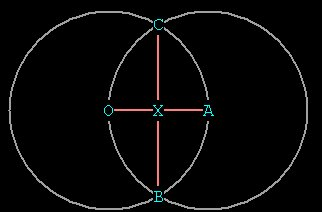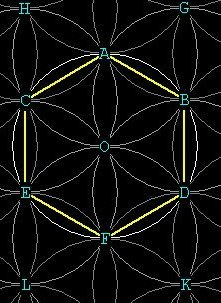MATHS  PAGE

Large vesica properties:Figure 1   --- A large vesica

Let the radius of each circle = 1.  Then notice OB = OA = OC = AC = AB = radius = 1.
Also notice CB bisects OA. So OX = CB = 1/2.
By Pythagorean Theorem, CX² = CA² - XA²  = 1 -   ¼  = 3/4.
So CX =   \/¯3 / 2.  CB = CX + XB so CX =  \/¯3/2 +  \/¯3/2 =  \/¯3.

Small vesica properties:Figure 2  ---- two views of the small vesica OA.  OA has been drawn with major and minor axes as with the large vesica above.

Notice that there is a sphere at C and one at B. We already know from above that the long axis of the large vesica (OCAB) is  \/¯3. The distance from C to W (located at  the right edge of the sphere centered at C) is 1, because it is the radius. Similarly for the distance BP, for  the sphere centered at B. A line AO will bisect a line CB, so that X  is the midpoint between C and B.
So we have :
C_________(_X_)_________B
P      W

distance CX = distance XB =  \/¯3/2. This is the same distance we found for CX of the large vesica  in Figure 1.
Distance CW = 1 because it's the radius of C.
Therefore distance XW = 1 -  \/¯3/2, or,   (2 -  \/¯3) / 2.

Distance PW  = twice  distance XW, so the short axis of the small vesica is 2 -  \/¯3.

So the ratio of the long axis to the small axis in the smaller vesica is:  1 / (2 -  \/¯3)
= 2 +  \/¯3.

(Note that (2 -  \/¯3) * (2 +  \/¯3) = 1. So multiplying both numerator and denominator by
2 +  \/¯3 gives 2 +  \/¯3).

Special characters:
\/¯  ² ³ × ½ ¼ Ø  \/¯(Ø² + 1)